Substance Transport Methodology

# Overview

## Abstract

The engine transporter was created to extract the methodology of substance transport from the individual systems. This creates a generic set of transport functions that can be used by any existing or future system by performing calculations on the circuits defined in the Common Data Model (CDM). At each time step, the mass, concentration, substance volume, volume fraction, and/or partial pressure are calculated based on the assumption that substances travel with the fluid flow.

## Introduction

Substances are transported through the body within the fluids, such as air in the Respiratory System and blood in the Cardiovascular System. To assess the substance levels of a patient, the substance must circulate through the system and distribute into the different nodes. The mass and concentration of a fluid must be calculated for substances in a liquid, and the volume of an individual substance (gas) and the volume fraction must be calculated for a gaseous fluid. After these basic calculations have been completed, calculations for partial pressures (gases), saturation (oxygen and carbon dioxide), and system totals (mass, concentration, volumes) are completed.

The engine modeling approach takes the human body and conceptually divides it into various fluid compartments that represents a real division in terms of how portions of the body's water, solutes, and suspended elements are segregated . Compartments can be further discretized into smaller sub-compartments with a hierarchical relationship as you drill into various systems. In the engine, compartments can be defined to encapsulate circuit nodes that allow easy organization, access, and synchronization of all system parts.

Links represent connections between compartments with a directional flow component defining a volume change each time-step. In the engine, links can be assigned a path that provides the instantaneous flow value. Figure 1 shows the base transport elements definitions in an example graph.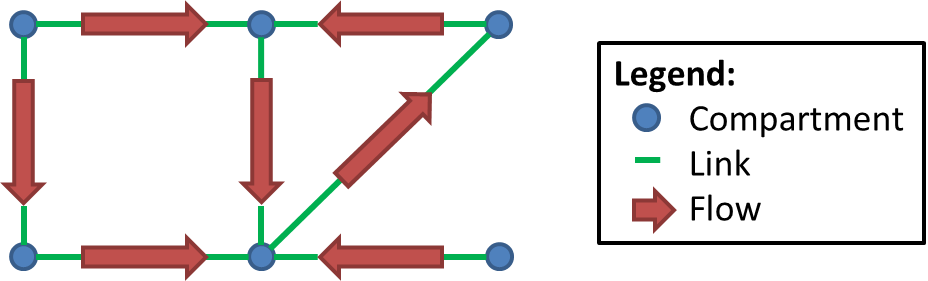Figure 1. This is a conceptual example graph that describes the lowest level elements used to define properties used by the Transporter. Links provide flow between compartments that store both fluid and substance quantity information.

# System Design

## Background and Scope

### Requirements

Given the overall engine goals, we set out to create a generic and reusable substance transporter. Some high-level requirements include:

• Generic - All systems should be able to use the same basic transporter engine. This allows rapid development, and makes engine outputs much easier to validate and verify.
• Computational Speed - The engine is required to maintain a transient full-body solution faster than real time on typical personal computers.
• Modular - Using the same basis for design and construction will aid in keeping the system decoupled.
• Extensible - We must take future growth into consideration and allow users and developers the proper tools and building blocks on which to add new functionality.
• Dynamic - Feedback mechanisms are required for each system. It is beneficial to be able to dynamically change, add, or remove compartments and links.
• Conservation - We must uphold sound scientific principles and conserve mass.
• Common Data Model - The entire solution must reside within and effectively use the Common Data Model and Software Framework.
• Fluid types - The engine will include liquid and gas systems. It is beneficial to use the same solver for both types.
• Bifurcations - Each compartment can have an unlimited number of links providing flow/substances both in (up stream) and out (down stream).
• Large flows - The transporter must be able to handle any size flows, including instances where significantly more volume is moved than exists in a given compartment for a given time-step.

### Approach

The Transporter is implemented generically for both liquid and gas systems using the same high-level definitions for substance properties, which are  :

• Extensive property: additive for independent, non-interacting subsystems - proportional to the amount of material in the system
• Property changes with amount change
• Pouring some out will change the value
• Examples: Mass, Volume, Length, Amount
• Intensive property: a bulk property, meaning that it is a physical property of a system that does not depend on the system size or the amount of material in the system
• Property doesn't change with amount change
• Defines and identifies substances
• Pouring some out will not change the value
• Examples: Concentration, VolumeFraction, and Temperature

The Transporter assumes that fluid movement (i.e. convection) has already taken place - generally calculated and updated inside the system by the circuit solver (Circuit Methodology). Once the convective fluid movement properties of compartment volume and link flow are updated for the current time-step being analyzed, the extensive and intensive substance values at each compartment can be determined by using the previous time-step state. Table 1 shows the parameters needed to calculate the advective transport by bulk flow.

Table 1. The variable definitions as well as the mapped property used to calculate advective transport.
Parameter Definition Liquid Variable Gas Variable
IC Current compartment intensive property Concentration Volume Fraction
ISC Source compartment intensive property Concentration Volume Fraction
fIL Input link flow Volumetric flow Volumetric flow
fOL Output link flow Volumetric flow Volumetric flow
t time-step Time Time
VC Current compartment volume Volume Volume
Eo,C Current compartment previous time-step extensive property Mass Substance volume

The instantaneous substance quantity values can be determined in each compartment of a graph by doing a mass balance calculation using Equation 1, where m is the mass on the current compartment and both min and mout are provided by links to any number of other compartments.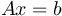Equation 1.

Equation 1 can be further broken out using the parameters in Table 1 to give Equation 2.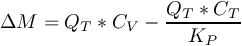Equation 2.

Rearranging Equation 2 gives Equation 3.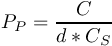Equation 3.

By simultaneously combining Equation 3 for all compartments in a graph, the linear equations can be written in the form of Equation 4 to solve for the new intensive properties throughout. A is the matrix of constants, x is the vector of all intensive properties, and b is the right side vector of known previous time-step extensive properties.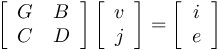Equation 4.

## Data Flow

The current implementation of the Circuit Transporter is set up to operate on the circuit nodes using the Preprocess, Process, and Post Process methodology of the other systems.

### Preprocess

The Circuit Transporter has no functionality in Preprocess.

### Process

The generic substance methodology developed for the engine is used to solve for the mass, concentration, substance volume, and volume fraction in each compartment each time-step. The steps used by the transporter to solve a graph using Equation 4 in a given time-step are:

1. Loop over compartments to populate the A matrix (one row per compartment) - this is the same for all substances
1. Handle infinite volume (often the environment) by setting intensive property constant
2. Handle no volume by using an approximate zero (1e-20) value to prevent a singular matrix
3. Handle source link flows (out of the compartment)
4. Handle target link flows (into the compartment)
2. Loop over all substances
1. Populate b vector with previous time-step masses
2. Solve for x vector intensive properties
3. Parse intensive properties and calculate new extensive properties

Each individual system uses the Calculate Substance Transport function to generically complete transport during the Process step.

### Post Process

The Post Process step moves everything calculated in Process from the next time-step calculation to the current time-step calculation. The Circuit Transporter has no specific Post Process functionality. Each system post-processes their circuit and moves the mass, concentration, substance volume, volume fraction, and partial pressure from "next" to "current".

# Results and Conclusions

## Verification

There are three successful unit tests that were created specifically to ensure the transporter is giving results as expected:

• Liquid Transport Test
• Gas Transport Test
• Large Flow Transport Test

## Conclusion

This generic methodology has proven to be a satisfactory algorithm for transport within the human body. The methodology is robust and successfully navigates all of the lumped parameter models in the engine. The algorithm is able to run faster than real time, allowing the engine to run faster than real time. Mass is conserved throughout the calculation.

# Appendices

## Data Model Implementation

SECompartmentTransportGraph

SESubstanceTransportAmount

SESubstanceTransportEdge

SESubstanceTransporter

SESubstanceTransportGraph

SESubstanceTransportVertex

## Acronyms

CDM - Common Data Model